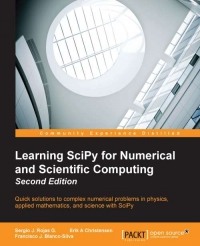# Learning SciPy for Numerical and Scientific Computing, 2nd Edition : Quick solutions to complex numerical problems in physics, applied mathematics, and science with SciPySciPy is an open source Python library used to perform scientific computing. The SciPy (Scientific Python) package extends the functionality of NumPy with a substantial collection of useful algorithms.

The book starts with a brief description of the SciPy libraries, followed by a chapter that is a fun and fast-paced primer on array creation, manipulation, and problem-solving. You will also learn how to use SciPy in linear algebra, which includes topics such as computation of eigenvalues and eigenvectors. Furthermore, the book is based on interesting subjects such as definition and manipulation of functions, computation of derivatives, integration, interpolation, and regression. You will also learn how to use SciPy in signal processing and how applications of SciPy can be used to collect, organize, analyze, and interpret data.
Read Source: Learning SciPy for Numerical and Scientific Computing, 2nd Edition : Quick solutions to complex numerical problems in physics, applied mathematics, and science with SciPy»